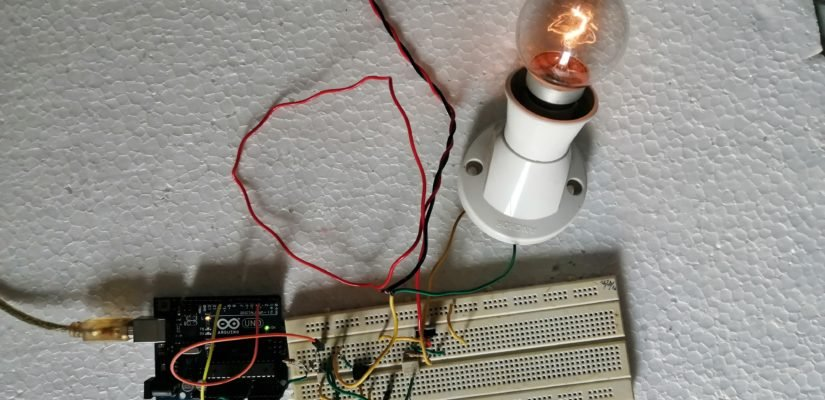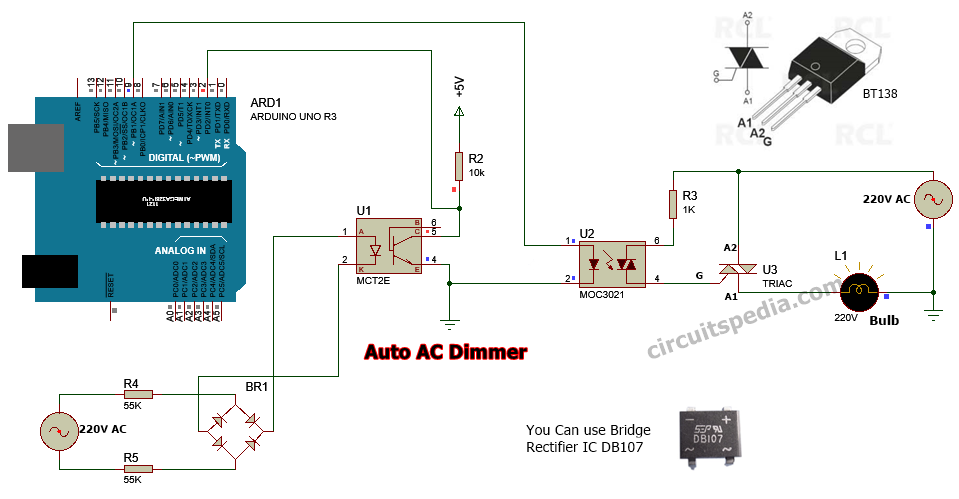## What is dimmer

Dimmer is a circuit that control the voltage level by changing its waveform and gives output minimum or less than the input and make the brightness of light dim or change the speed of fan as required level.

AC dimmer is mainly two types, one is manually and second is automatic. In manually dimmer circuit dimming can be created using a adjusting of moving knob of a Potentiometer or any variable resistor as required output voltage level. In automatic Dimmer the dimming effect created using a predefined level and time duration.

Automatic dimmer can be used in any AC decorative lights for getting an special effect and  for automation of devices. This one channel ac dimmer made using arduino UNO board using a specified coding.

Ac dimmer circuit made by using Triac and optocoupler .  A zero cross detector circuit is required for ac dimming purpose . This provides a stable signal at each zero level of wave form and send a signal to arduino to know about each zero level of sine wave form.

Triac is firing for microseconds on each pulses continuously. Pin 2 of digital pin in arduino is an interrupt pin that is used here for providing a delay in firing the triac. Then the output will create as dimming signal by cutting edge of a part of wave form of sine .

Note - This circuit uses 220v ac so this is very dangerous. So very careful when test. If you not sure about working with ac supply then don't test itself because it can cause of death.Components Required

Bridge rectifier ic DB107 - 1

Optocoupler IC MCT2E or 4N35 - 1

Optotriac IC MOC3021 - 1

Triac BT136 or BT138 - 1

Arduino UNO Board - 1

Resistors

56K- 2

10K-1

1K -1

This is one channel auto ac triac dimmer using arduino uno. You can connect any resistive load with this upto 200W easily. Any decorative light can conneted for special effect.

```Code
/*AC Light Control

Changed zero-crossing detection to look for RISING edge rather
than falling.  (originally it was only chopping the negative half
of the AC wave form).

Also changed the dim_check() to turn on the Triac, leaving it on
until the zero_cross_detect() turn's it off.

#include  <TimerOne.h>          // Avaiable from http://www.arduino.cc/playground/Code/Timer1
volatile int i=0;               // Variable to use as a counter volatile as it is in an interrupt
volatile boolean zero_cross=0;  // Boolean to store a "switch" to tell us if we have crossed zero
int AC_pin = 9;                // Output to Opto Triac
int dim = 0;                    // Dimming level (0-128)  0 = on, 128 = 0ff
int inc=1;                      // counting up or down, 1=up, -1=down

int freqStep = 75;    // This is the delay-per-brightness step in microseconds.
// For 60 Hz it should be 65
// It is calculated based on the frequency of your voltage supply (50Hz or 60Hz)
// and the number of brightness steps you want.
//
// Realize that there are 2 zerocrossing per cycle. This means
// zero crossing happens at 120Hz for a 60Hz supply or 100Hz for a 50Hz supply.

// To calculate freqStep divide the length of one full half-wave of the power
// cycle (in microseconds) by the number of brightness steps.
//
// (120 Hz=8333uS) / 128 brightness steps = 65 uS / brightness step
// (100Hz=10000uS) / 128 steps = 75uS/step

void setup() {                                      // Begin setup
pinMode(AC_pin, OUTPUT);                          // Set the Triac pin as output
attachInterrupt(0, zero_cross_detect, RISING);    // Attach an Interupt to Pin 2 (interupt 0) for Zero Cross Detection
Timer1.initialize(freqStep);                      // Initialize TimerOne library for the freq we need
Timer1.attachInterrupt(dim_check, freqStep);
// Use the TimerOne Library to attach an interrupt
// to the function we use to check to see if it is
// the right time to fire the triac.  This function
// will now run every freqStep in microseconds.
}

void zero_cross_detect() {
zero_cross = true;               // set the boolean to true to tell our dimming function that a zero cross has occured
i=0;
digitalWrite(AC_pin, LOW);       // turn off TRIAC (and AC)
}

// Turn on the TRIAC at the appropriate time
void dim_check() {
if(zero_cross == true) {
if(i>=dim) {
digitalWrite(AC_pin, HIGH); // turn on light
i=0;  // reset time step counter
zero_cross = false; //reset zero cross detection
}
else {
i++; // increment time step counter
}
}
}

void loop() {
dim+=inc;
if((dim>=128) || (dim<=0))
inc*=-1;
delay(60);
}

Also read Distance measurement using arduino

```